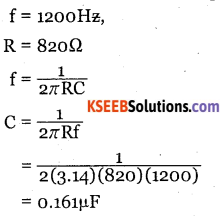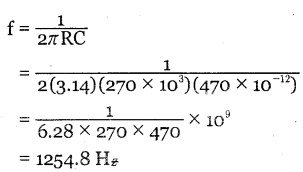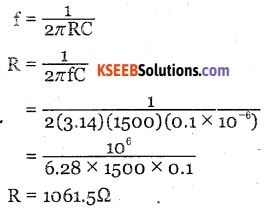2nd PUC Electronics Question Bank Chapter 6 Oscillators

Students can Download 2nd PUC Electronics Chapter 6 Oscillators Questions and Answers, Notes Pdf, 2nd PUC Electronics Question Bank with Answers helps you to revise the complete Karnataka State Board Syllabus and score more marks in your examinations.

Karnataka 2nd PUC Electronics Question Bank Chapter 6 Oscillators

2nd PUC Electronics Oscillators One Mark Questions and Answers

Question 1.
What is a positive feedback?
The process of applying a portion of the output in phase with the input is called positive feedback.

Question 2.
What is an oscillator?
Oscillator is a circuit that converts DC energy into an AC energy of desired frequency.

Question 3.
What are sinusoidal oscillators?
Oscillators that produce sinusoidal waveforms are called sinusoidal oscillators.

Question 4.
What are non-sinusoidal or relaxation oscillators?
Oscillators that produce non-sinusoidal waves like triangular wave, square wave or saw tooth waves are called non-sinusoidal oscillators.Question 5.
What are damped oscillations?
Damped oscillations are electrical oscillations whose amplitude keeps on decreasing with time but frequency remains a constant.

Question 6.
What are undamped oscillations?
Undamped oscillations are electrical oscillations whose amplitude remains constant with respect to time.

Question 7.
Write the expression for the voltage gain of a positive feedback amplifier.
Voltage gain with positive feedback,
$$A_{ f }=\frac { A }{ 1-A\beta }$$

Question 8.
Draw the basic block diagram of an oscillator.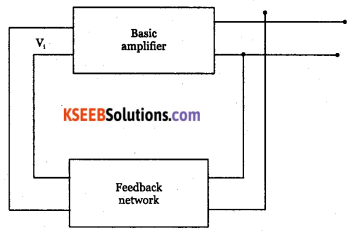Question 9.
What is a tank circuit?
Tank circuit or oscillatory circuit is a circuit that produces electrical oscillations of any desired frequency.

Question 10.
What should be value of loop gain to start oscillations?
The loop gain Ap should be greater than 1 to start the oscillations.

Question 11.
What should be the value of loop gain for sustained oscillations?
The loop gain Ap should be equal to 1.Question 12.
How many RC branches are required for a phase shift oscillator?
Three.                                                                ‘

Question 13.
What is the amount of phase shift in each RC section of a phase shift oscillator?
60°.

Question 14.
Write the expression for frequency of oscillation of a Wein bridge oscillator.
Frequency,
$$\mathrm{f}=\frac{1}{2 \pi \mathrm{RC}}$$

Question 15.
Write the expression for frequency of oscillation of an RC phase shift oscillator.
$${ f }=\frac { 1 }{ 2\pi \sqrt { 6 } RC } \quad { or\quad }{ f }=\frac { 0.065 }{ { RC } }$$

Question 16.
Write the expression for the frequency of osillations of a Collpitts oscillator
Frequency $${ f }=\frac { 1 }{ 2\pi \sqrt { { LC }_{ s } } } { where\quad }{ C }_{ s }=\frac { { C }_{ 1 }{ C }_{ 2 } }{ { C }_{ 1 }+{ C }_{ 2 } }$$

Question 17.
Write the expression for the frequency of oscillation of a Hartley oscillator.
Frequency $${ f }=\frac { 1 }{ 2\pi \sqrt { { LC }_{ s } } }$$ where L = L1 + L2

Question 18.
Write the expression for frequency of oscillation of a crystal oscillator.
Frequency $${ f }=\frac { 1 }{ 2\pi \sqrt { { LC }_{ s } } }$$

Question 19.
Does the bridge circuit produce any phase shift in a Wein bridge oscillator?
No. The bridge circuit does not produce any phase shift.Question 20.
Write the expression for the feedback factor of colpitts oscillator.
Feedback factor, $$\beta=\frac{C_{1}}{C_{2}}$$

Question 21.
Write the expression for the feedback factor of Hartley oscillator.
Feedback factor, $$\beta=\frac{L_{2}}{L_{1}}$$

Question 22.
Write the expression for the feedback factor of phase shift oscillator.
Feedback factor, $$\beta=\frac{R_{i}}{R_{f}}=\frac{1}{29}$$

Question 23.
Write the expression for feedback factor of wein bridge oscillator.
Feedback factor, $$\beta=\frac{1}{3}$$

Question 24.
Mention the principle used in crystal oscillator.
Piezo-electric effect.

Question 25.
What is the type of feedback used in Colpitts oscillator?
Capacitive feedback.Question 26.
What is the type of feedback used in Hartley oscillator?
Inductive feedback.

Question 27.
Draw the symbol of crystal.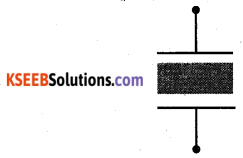Question 28.
Draw the pin diagram of 555 timer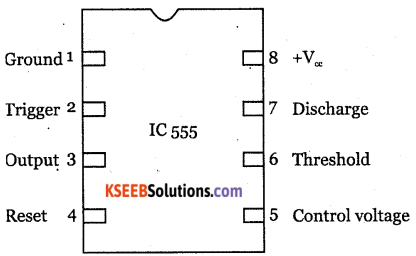2nd PUC Electronics Oscillators Two Marks Questions and Answers

Question 1.
Distinguish between sinusoidal and relaxation oscillators.
Sinusoidal oscillators produce sinusoidal wave forms where as relaxation oscillators produce non-sinusoidal wave forms like triangular wave, square wave or rectangular wave.Question 2.
Distinguish between damped and undamped oscillations.
Damped osillations are the electrical oscillations whose amplitude goes on decreasing with respect to time. Undamped oscillations are electrical oscillations whose amplitude remains a constant.

Question 3.
Explain the conditions of Barkhaussan criterion.

• The total phase shift around the closed loop should be 0° or 360°.
• The magnitude of product of the open loop gain of the amplifier (A) and feedback ratio (P) is unity. Ie, | Aβ |= 1 .

Question 4.
Draw the circuit diagram of Hartley oscillator.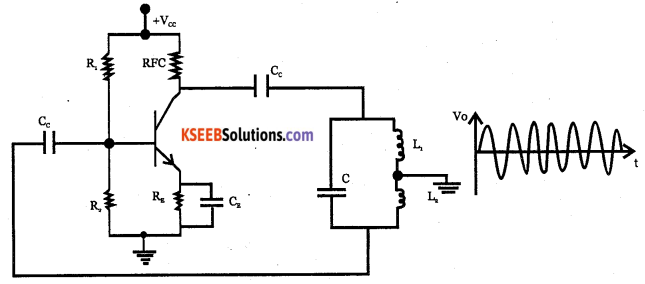Question 5.
Draw the circuit diagram of a Colpitts oscillator.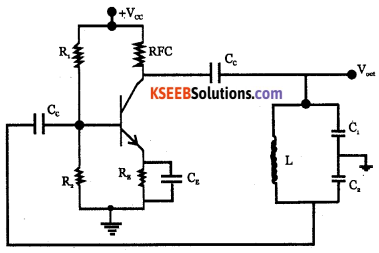Question 6.
Draw the circuit diagram of RC phase shift oscillator.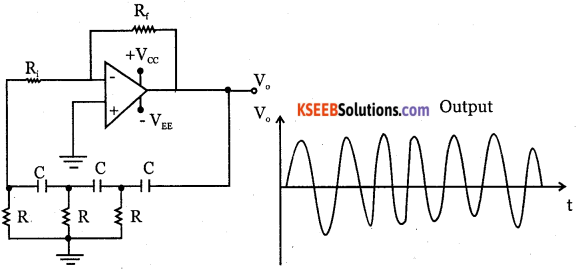Question 7.
Draw the circuit diagram of Wein bridge oscillator.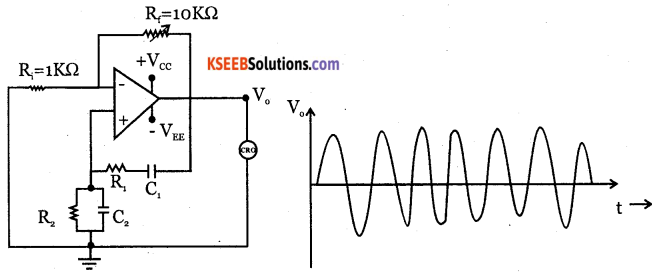Voltage gain A = $$1+\frac{\mathrm{R}_{3}}{\mathrm{R}_{4}}=3$$

Question 8.
Draw the circuit diagram of a crystal oscillator.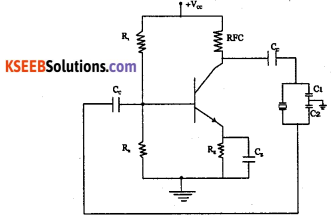Question 9.
Write any two applications of Hartley oscillator.
Hartley oscillator is used inQuestion 10.
Mention any two applications of Colpitts oscillator.
Collpitts oscillator is used in:

Question 11.
Mention any two advantages of a crystal oscillator over LC and RC oscillators.

• They have high frequency stability.
• They are used as a reference for measurement and comparison of frequencies.

Question 12.
Mention the limitations of LC oscillators.
The value of resistors and inductors change due to changes in temperture. Hence, the
frequency of LC and RC oscillator do not remain constant.

Question 13.
Write any two advantage of RC oscillators over LC oscillators.

• RC oscillators provide constant output and good stability.
• RC oscillators do not require any inductor and hence size and cost get reduced.

Question 14.
Mention any two applications of a crystal oscillator.
Crystal oscillators are used in communication transmitters and receivers, in digital watches and calculators.Question 15.
Draw block diagram of 555 timer.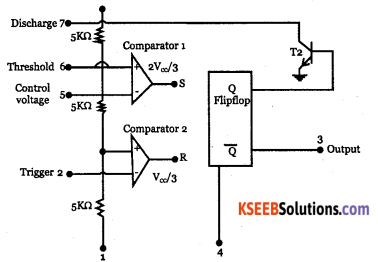Question 16.
Draw the diagram of bistable multivibrator.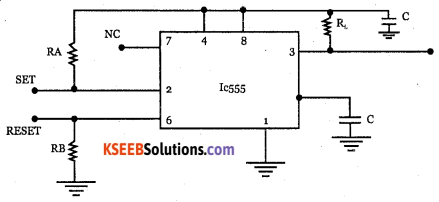2nd PUC Electronics Oscillators Three Marks Questions and Answers

Question 1.
Explain briefly how oscillations are produced in a tank circuit.
Tank circuit or oscillatory circuit is a circuit which produces electrical oscillations of any desired frequency. A simple oscillatory circuit has a capacitor C and inductance L in parallel.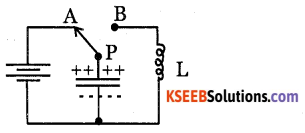When P is connected to A the capacitor gets charged to a potential V of the battery, with upper Plate being positive and lower plate negative.When p is conncted to B, the capacitor starts discharging through inductor L. when capacitor is fully discharged, the energy of magnetic field in the coil will be maximum.

Once capacitor is discharged, the magnetic field will begin to collapse and produce a counter emf. This keeps the current to flow in the opposite direction and the capacitor now gets charged with opposite polarity, making upper plate of capacitor negative and lower plate positive.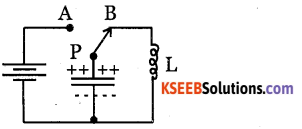After the collapsing magnetic field has recharged the capacitor, the capacitor now begins to discharge hence current now flows in the opposite direction. The interchange of energy between L and C is repeated again in the production of oscillations.

The frequency of oscillations generated by the LC tank circuit depends on values of L and C, given by  $$\mathrm{f}=\frac{1}{2 \pi \sqrt{\mathrm{LC}}}$$Question 2.
With a circuit diagram, explain the principle of phase shift in the RC circuit.Each RC circuit is adjusted for 60° phase shift. Hence the total phase shift from feedback network is 180°. The additional 180° phase shift is introduced by op-amp inverting amplifier. As a result, phase shift around the loop will be 360°, satisfying the required condition for the positive feedback and the circuit acts as an oscillator.

Question 3.
With the circuit diagram, explain the principle of lead-lag network.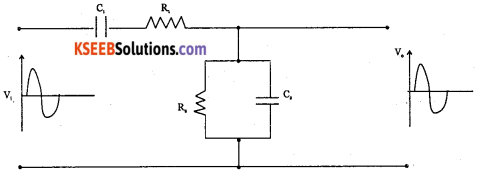A circuit in which output voltage leads or lags the input voltage is called a lead lag network. It has series combination of R1 and C1 and a paralel combination of R2 and C2. At low frequencies, series capacitor C1 looks open and there is no output. At high frequencies, C2 acts as short and no output is obtained. At some frequency f output is maximum if R1= R2 = R and C1= C2 =C, then frequency $$\mathrm{f}=\frac{1}{2 \pi \mathrm{RC}}$$

At low frequencies, phase angle is positive and the circuit acts as a lead network. At high frequencies, phase angle is negative and circuit acts as a lag network.                          ‘

Question 4.
Draw the circuit diagram of a Hartley oscillator. Mention the expression for the frequency of oscillations.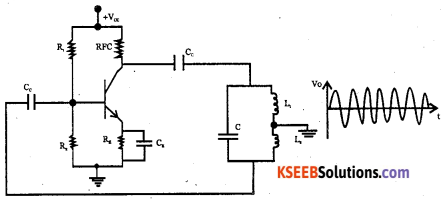Frequency $${ f }=\frac { 1 }{ 2\pi \sqrt { { LC } } } { Where }{ C }=\frac { { C }_{ 1 }{ C }_{ 3 } }{ { C }_{ 1 }+{ C }_{ 2 } }$$

Question 5.
Draw the circuit diagram of Colpitts oscillator. Mention the expression for its feedback factor.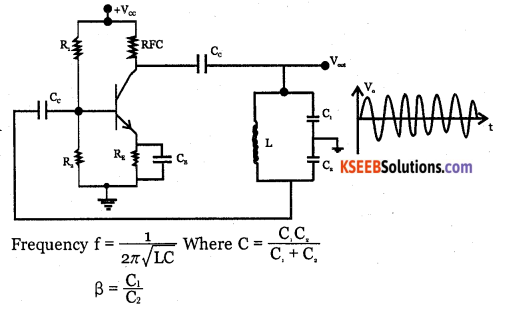Question 6.
Draw the circuit diagram of RC phase shift oscillator. Draw its output waveform.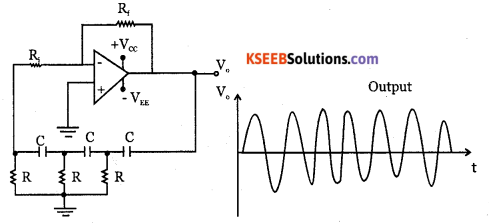Question 7.
Draw the circuit diagram of wein bridge oscillator. Mention the expression for its voltage gain.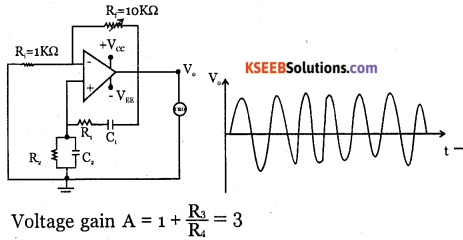Question 8.
Draw the circuit diagram of crystal oscillator. Draw the electrical equivalent circuit of a crystal.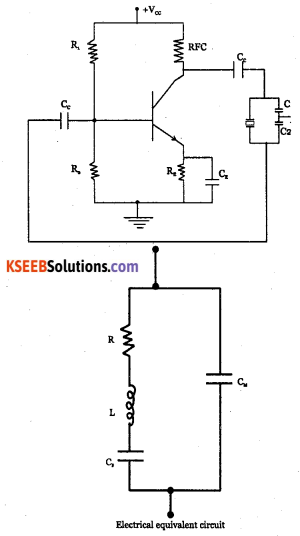2nd PUC Electronics Oscillators Five Marks Questions and Answers

Question 1.
With a circuit diagram, explain the working of Hartley oscillator. Write the expression for its frequency and feedback factor.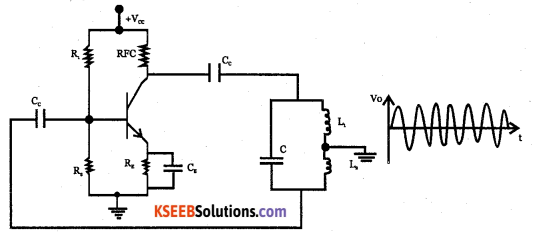Resistors R1R2 and RE provide the required DC bias to the transistor. CC is coupling capacitor and CE is a bypass capacitor. RFC is radio frequency choke which isolates power supply from the oscillations at the collector. Inductors L1, L2 with capacitor C forms the tank circuit. Frequency of oscillations
$$f=\frac{1}{2 \pi \sqrt{L C}}$$
Where L = L1 + L2

When power supply is switched on, collector current increases and charge the capacitor. When the capacitor is fully charged, it discharges through L1 and L2 and oscillations will be set up. The voltage across L2 is feedback to amplifier circuit and that across L1 is taken as output. 180° phase shift is provided by the transistor amplifier and another 180° phase shift is provided by the tank circuit. Thus totally 360° phase shift is obtained, which is the required condition for ‘ the sustained oscillations.
The voltage feedback ratio  $$\beta=\frac{\mathrm{L}_{2}}{\mathrm{L}_{1}}$$Question 2.
Draw the circuit diagram of colpitts oscillator and explain its action. Write the expression for its frequency and feedback factor.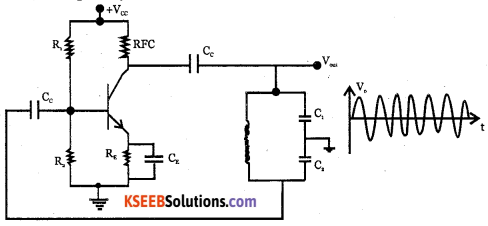The resistors R1 R2 and RE provide necessary DC bias to the transistor. CC is the coupling capacitor and CE is a bypass capacitor, RFC is radio frequency choke which prevents high frequency oscillations reaching the DC power supply. The tank circuit has an inductor and two capacitor C1 and C2 in series.

When power supply is switched on, the increasing collector current charges C1 and C2. The potential across the two capacitors are in opposite phase. When the capacitors are fully charged, voltage across C2 is fed back to amplifer circuit. The amplifier produces a phase shift of 180° and the tank circuit produces a phase difference of 180°. Hence the total phase shift is 360°, which is the condition required for the sustained oscillations.

The feedback fraction is $$\beta=\frac{C_{1}}{C_{2}}$$, and the frequency of oscillations produced is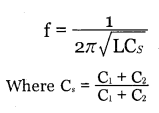Question 3.
With a circuit diagram, explain the action of a RC phase shift oscillator. Write the expression for its frequency and feedback factor.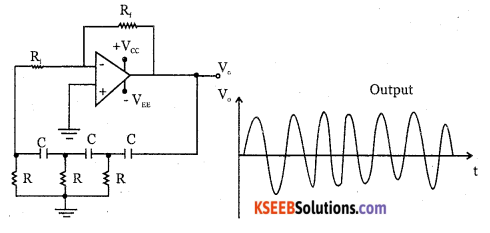It has op-amp as the amplifying stage and three RC cascaded RC network as the feedback circuit. The op-amp is used as an amplifier. The feedback circuit provides feedback voltage from the output back to the input of the amplifier. The op-amp is used in the inverting mode. Thus, op-amp gives a phase shift of 180° at the output. An additional 180° phase shift required for oscillations is provided by the 3 RC networks.

When the power supply is switched on, the noise voltage induced between the pin numbers 2 and 3 is amplified by the op-amp producing a phase shift of 180°. The output is fed to RC phase shift network which introduces a total phase shift of 180° by charging and discharging action of a capacitor through the resitors. Each RC network produces a phase shift 60° and hence three RC
$$f=\frac{1}{2 \pi R C \sqrt{6}}=\frac{0.065}{R C}$$
The feedback fraction is β = $$\beta =\frac { R_{ i } }{ R_{ f } } =\frac { 1 }{ 29 }$$Question 4.
Draw the circuit diagram of Wein bridge oscillator and explain its working. Write the expression for the frequency and feedback factor.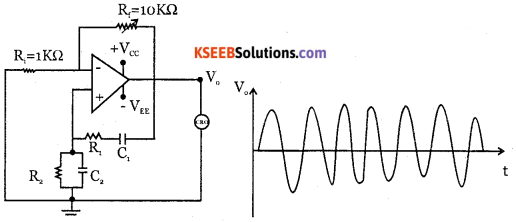The circuit diagram shows wein bridge oscillator in which wein bridge is connected between amplifier input terminal and the output terminal. The bridge has a series RjC, network in one arm and a parallel R2C, network in the adjoining arm. In the remaining arm of the bridge, resistors R3, R4 are connected. The phase angle criterion for oscillation is that total phase shift around the circuit should be 0°.

This is possible only when output is fedback to the non­inverting input without any phase shift since the lead-lag network does not produce any phase shift. This condition occurs only when the bridge is balance i.e., at resonance. The frequency of oscillation is given by $$f=\frac{1}{2 \pi R C}=\frac{0.159}{R C}$$
The feedback fraction, β = $$\frac{1}{3}$$

Question 5.
With a circuit diagram, explain the working of crystal oscillator. Write the expression for
its frequency of oscillation.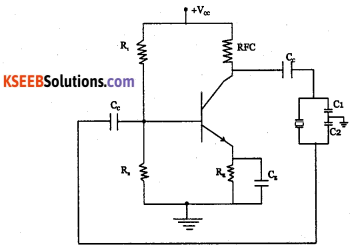Crystal oscillator works on the principle of piezo electric effect. According to piezo electric effect, when AC voltage is applied across the crystal, it vibrates at the frequency of the applied voltage. If the mechanical force is applied to make the crystal vibrate, then an AC voltage is generated across it. Quaertz, Rochelle salt and Tourmaline show this phenomena of piezo electric effect.

The resistors R1, and R2, and RE form voltage divider stabilised dc bias circuit. The radio frequency choke prevents oscillations produced from entering the dc power supply.

When the circuit is switched on, the noise signal generated increases the collector current which charges the capacitors C1, and C2. The discharging current induces an emf across plates of the crystal which oscillates and produces the resonant frequency. The crystal, C1, and C2 produce a phase shift of 180° and the transistor produces a phase shift of 180°. Thus the phase shift around the loop is 360°, the require condition for the sustained oscillations.

The frequency of oscillations produced is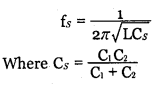The frequency stability of the crystal oscillator is very high because frequency of oscillation is unaffected by changes in supply voltage and transistor parameters.Question 6.
Explain briefly each part of block diagram of 555 timer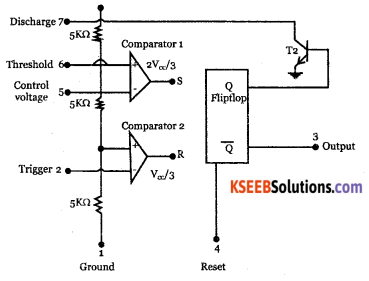It has two comparators, an RS flip flop, npn transistor and three 5KΩ resistors. Resistive network has three equal resistors and acts as a voltage divider.

When the threeshold voltage is greater than the control voltage, the comparator will set the flip flop and its output is HIGH. HIGH output from the flip flop saturates the discharge transistor T. The complementary output of the flip flop going to pin 3 is low. The condition will remain until comparator 2 triggers the flip flop even if the voltage at the threshold input falls below $$\frac{2}{3}Vcc$$ ie, comparator 1 can not couse flip flop to change again comparator 1 can only force the 3 flip flops output HIGH.

To change output of the flip flop to low’ state, the voltage at the input should fall below $$\frac{1}{3}Vcc$$

The comparator 2 triggers flip flop output to low state. The output from flip flop turns the discharge transistor T2 off. The output at pin 3 is high.

Problems with Solutions

Question 1.
A transistor Colpitts oscillator has L= 4mH, C1 =10nF, C2=10nF. Determine the frequency of oscillation.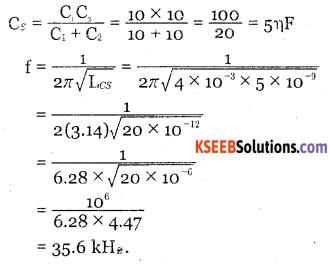Question 2.
A Colpitts oscillator circuit is to generate a frequency of 24 kHz. The capacitors used are Cf= 0.2 μF, C2=0.22μF. Find the value of inductor used.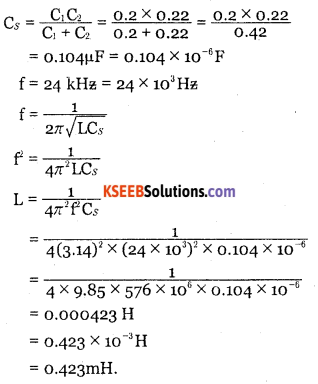Question 3.
A colpitts oscillator oscillates at 1.13MHz. If the inductor in the feedback network has a value of 20pH. and one of the capacitors value is 0.1 μF, calculate the value of the other capacitor.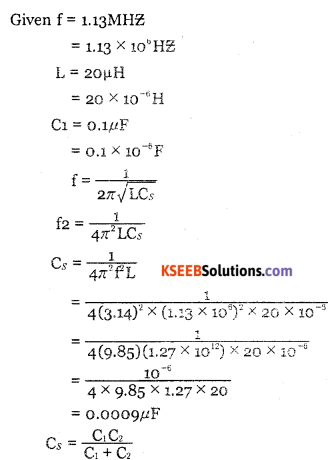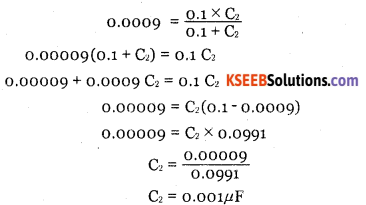Question 4.
Calculate the frequency and feedback ratio of the circuit given below.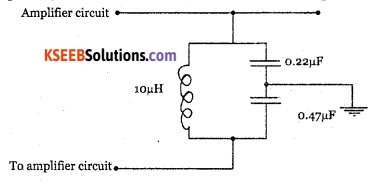Question 5.
A Hartley oscillator has L1=3mH, L2=5mH and C=10nF. Determine the frequency of oscillations.
L=L1+ L2 = 3 + 5 = 8mH
C = 10 ηf = 10 x 10-9 F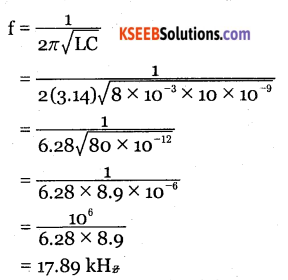Question 6.
A Hartley oscillator circuit is to generate a frequency of 1200 kHz. If the capacitor in the feedback network has a value of 220 pF and one of the inductor’s value is 20pH, calculate the other inductor.Question 7.
Hartley oscillator oscillates at 54 kHz. The inductors used have inductances L1 = 30mH and L2= 60mH. Find the value of capacitance of the capacitor
f = 54 kHz
= 54 x 103 Hz
L1= 30mH = 30 x 10-3H
L2= 6omH = 60 x 10-3 H
L = L1 + L2 = 30 + 60 = 90mH = 90 x 10-3H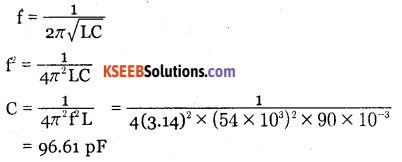Question 8.
Calculate the frequency and feedback ratio of the circuit given below.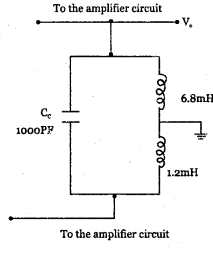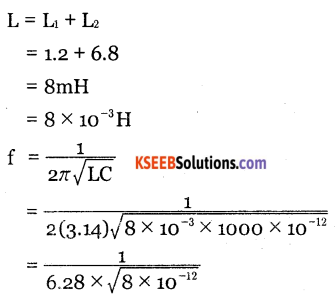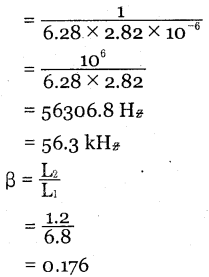Question 9.
RC Phase shift oscillator uses three identical RC sections in the feedback network. The values of the components are R=680Ω and C=220nF. Determine the frequency of oscillation.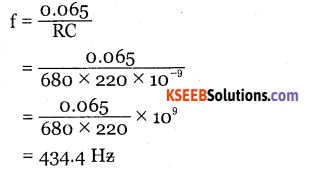Question 10.
What should be the value of capacitor required for a phase shift oscillator to produce a frequency of 338 Hz, if the resistance used is 220Ω.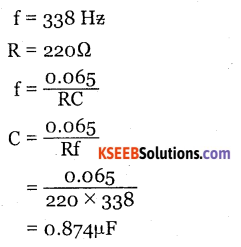Question 11.
The frequency of phase shift oscillator is 125 Hz. If the value of the capacitance of the capacitor used is 0.22pF, calulate the value of resistance used.
f = 125Hz
C = 0.22μf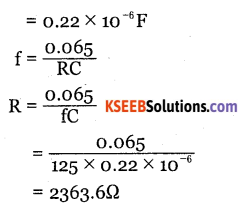Question 12.
The frequency of Wein bridge oscillator is 1200 Hz. If the resistance value used is 820Q calculate the value of the capacitance used.